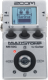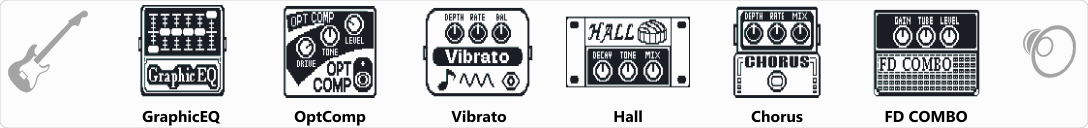# 70's Glam

Discussion in 'Zoom MS-50G' started by John Cope, Jun 15, 2022.

1. 70's GlamDevice: Zoom Ms-50g
Firmware: 3.10

Name on device: Glam
Optimized for: Guitar Amp

Effects chain:Glam sound of the late 70's

Effect: "GraphicEQ" (Dynamics / Filter), active - "yes"
"160Hz" = -1
"400Hz" = -5
"800Hz" = -3
"3.2kHz" = 6
"6.4kHz" = 6
"12kHz" = 7
"Level" = 100

Effect: "OptComp" (Dynamics / Filter), active - "yes"
"Drive" = 8
"Tone" = 62
"Level" = 61

Effect: "Vibrato" (Modulation / Sfx), active - "yes"
"Depth" = 78
"Rate" = 8
"Bal" = 72
"Tone" = 6
"Level" = 120

Effect: "Hall" (Delay / Reverb), active - "yes"
"Decay" = 10
"Tone" = 5
"Mix" = 46
"PreD" = 49
"Level" = 100
"Tail" = Off

Effect: "Chorus" (Modulation / Sfx), active - "yes"
"Depth" = 36
"Rate" = 21
"Mix" = 37
"Tone" = 4
"Level" = 100

Effect: "FD COMBO" (Amp simulator), active - "yes"
"Gain" = 24
"Tube" = 43
"Level" = 87
"Trebl" = 51
"Middl" = 45
"Bass" = 44
"Prese" = 52
"CAB" = FD COMBO 2x12
"OUT" = LINE

Note: This is a patch file, you will need to download and install the ToneLib-Zoom software to use the patch.

File size:
419 bytes
Views:
152